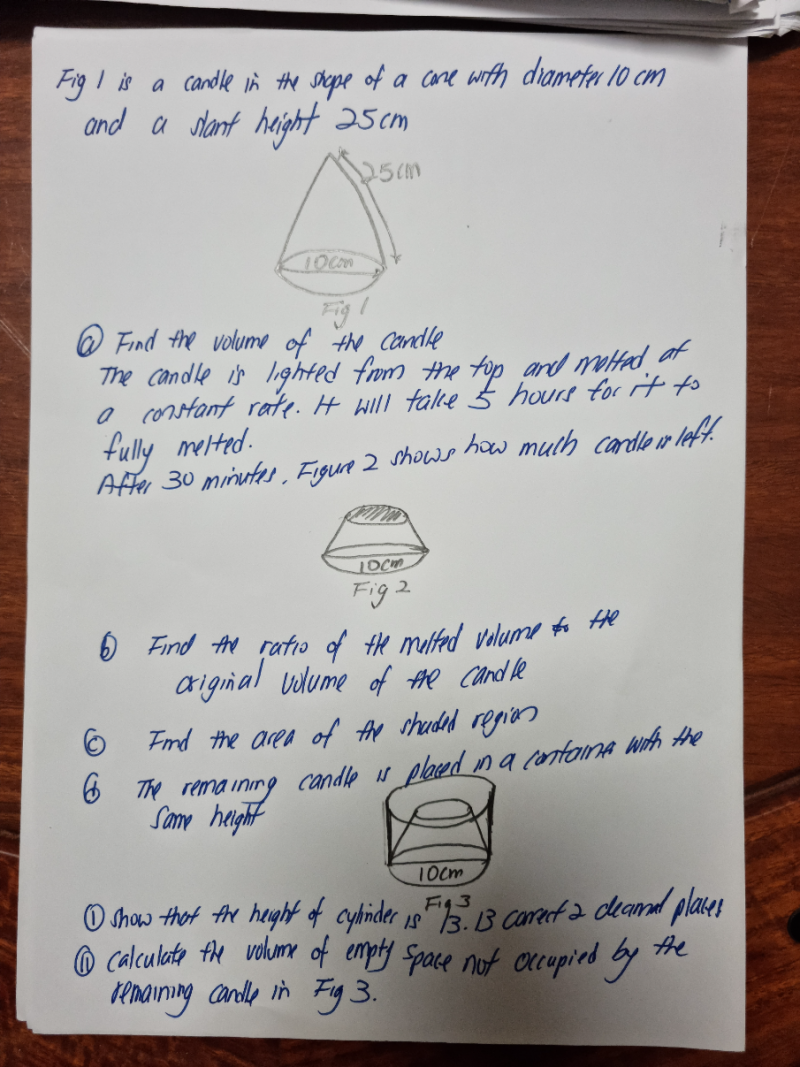# QuestionThank you.

(a)  Using Pythagoras theorem, height of cone = sqrt [(25)2 – (5)2 ] = sqrt(600)

Volume of cone =  (1/3)πr2h

Volume of conical candle = (1/3)π (5) sqrt(600) = 641.27 cm (to 2 dec. places)

(b) 300 mins —> 641.27
In 30 mins, melted volume of candle = (641.27/300) x 30 = 64.127
Melted volume of candle / Original Candle  = 64.127/641.27 = 0.1 or  (1/10)

(c)  Ratio of volumes of similar cones = (a/b) 3   where a and b are either both radius or both height of similar cones.
Ratio of  surface areas of similar cones = (a/b) 2   where a and b are either both radius or both height of similar cones.
So,  SA of shaded region of figure 2 =  [cube-root (1/10)]2 x SA of original conical candle
= (1/10)2/3  x  π  x (5)2
=  16.92 cm2

(d)  From (c),  π( r1)2 = (1/10)2/3  x  π  x (5)
( r1)2 =  (1/10)2/3  x  (5)
We know that for similar cones, the volume ratio is:
(1/3)π( r1)2h1  /   (1/3)π( r2)2h2 = 1/10

So,  h1 = [ (1/10) x  ( r2)2h2 ] / ( r1)2
=   [ (1/10) x (5)2 sqrt(600) ]  /  (1/10)2/3  x  (5)2
=   11. 3695

Hence height of cylinder = height of remaining conical candle =  sqrt(600) – 11.3695
= 13.13   (to 2 decimal places)

(e)   Volume of remaining conical candle = 641.27-64.127 = 577.143
Volume of cylinder =  πr2h = π x (5)2 x (13.13)
Hence Volume of empty space in fig 3 = Vol of cylinder – Vol of remaining conical candle
= π x (5)2 x (13.13)  –  577.143
=  454.08 cm2 (to 2 decimal places)